2019 Summer Math Practice Gr. 7 - Statistics and Probability
Complete all questions and submit at end. This is part of your summer math packet. All 5 parts must be completed by September 3, 2019. These questions are to reinforce statistics and probability from 6th grade.
Please do not use a calculator.
When answering please submit just a numerical answer.
Last Name *
Your answer
First Name *
Your answer
6th Grade Cluster *
Your answer
The data below are the number of text messages you send a day. Find the mean of the data. *
10, 12, 20, 8, 6, 10, 18
Your answer
The data below are the number of text messages you send a day. Find the median of the data. *
10, 12, 20, 8, 6, 10, 18
Your answer
The data below are the number of text messages you send a day. Find the mode of the data. *
10, 12, 20, 8, 6, 10, 18
Your answer
The data below are the number of text messages you send a day. Find the range of the data. *
10, 12, 20, 8, 6, 10, 18
Your answer
How do you calculate the mean? *
The absolute value is always ____________. *
What does MAD mean? *
Using the histogram "Student Attendance", which interval contains the most data values? *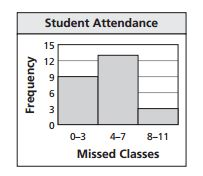What is the percent of students who missed 8 or more classes? *
Refer to "Student Attendance"Find the mean absolute deviation of the data for "Height". Round your answer to the nearest tenth, if necessary. *
Step 1: Find the mean of the data. Step 2: Find the absolute distance between each data value and the mean. Step 3: Find the sum of the absolute distances in Step 2. Step 4: Divide the sum in Step 3 by the total number of data values.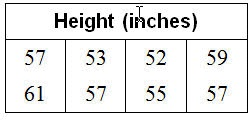Your answer
How many students responded to the "Number of Pets" question? *Your answer
What is the mean of the data? *
Refer to "Number of minutes studied"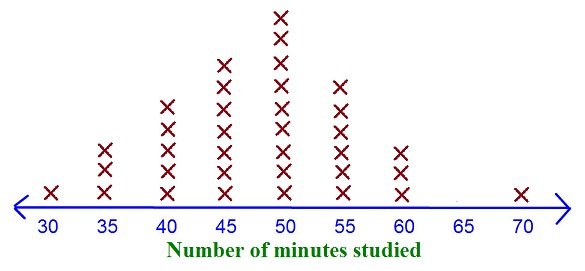Your answer
Is there an outlier? *
Refer to "Number of minutes studied"What is the peak of the data? *
Refer to "Number of minutes studied"Your answer
What is the range of magnitude? *
Refer to "Rat Island Data Chart"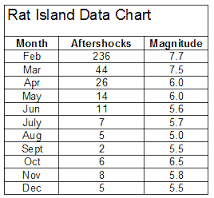Your answer
What is the average magnitude? *
Refer to "Rat Island Data Chart"Your answer
What was the average score on the science test? *
Refer to "Stem and Leaf Plot - Scores on Science Test"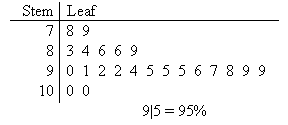Your answer
What was the median score on the science test? *
Refer to "Stem and Leaf Plot - Scores on Science Test"Your answer
What was the range on the science test? *
Refer to "Stem and Leaf Plot - Scores on Science Test"Your answer
The box-and-whisker plot represents the closing times of businesses in a town. What percent of the businesses close at 10 P.M. or later? *
Refer to "Closing Time"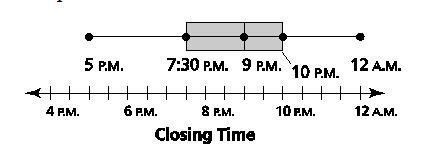Never submit passwords through Google Forms.
This form was created inside of Peabody Public Schools. Report Abuse - Terms of Service